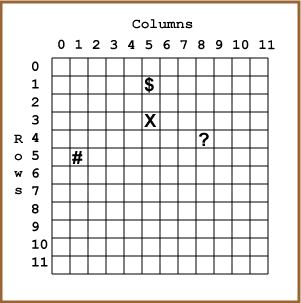The square with the X is column 5 row 3.

# Columns and Rows

Here is that graph paper again, now with more squares marked:The position of each square is given by its column number and row number. For instance, the square with the # is column=1 row=5. The square with the \$ is column=5 row=1. Notice that you have to be careful to get column and row in the right order.

### QUESTION 4:

What is the column and row of the square marked with ? ?